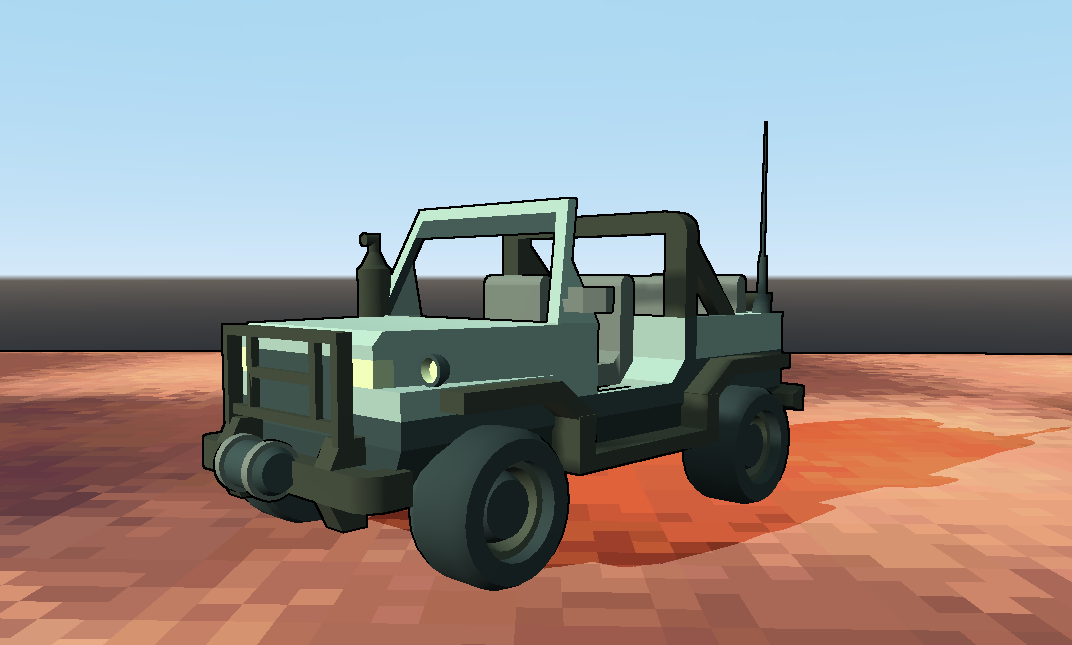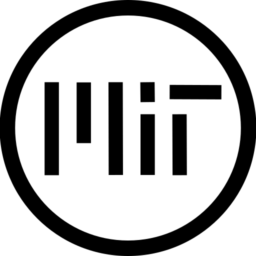# Post Effect Outline Shader for GLES2

Post effect outline shader. Create a quadmesh and set its size for x and y to 2. The position and rotation of the quadmesh is unimportant because it will be changed by the shader.

``````/*

*/

uniform int _thickness : hint_range(0,3) = 1;
uniform float _detail : hint_range(0.0,256.0) = 256.0;

varying mat4 CAMERA;

void vertex()
{
POSITION = vec4(VERTEX, 1.0);
CAMERA = CAMERA_MATRIX;
}

void fragment()
{
float depth = texture(DEPTH_TEXTURE, SCREEN_UV).x;
vec3 ndc = vec3(SCREEN_UV, depth) * 2.0 - 1.0;
vec4 view = INV_PROJECTION_MATRIX * vec4(ndc, 1.0);
view.xyz /= view.w;
float linear_depth = -view.z;

float pix;
for( int y=0; y<3; y ++ ) {
for( int x=0; x<3; x ++ ) {
pix[y*3+x] = texture( SCREEN_TEXTURE, SCREEN_UV + vec2( float( x-1 ), float( y-1 ) ) *  float(_thickness) / VIEWPORT_SIZE ).r;
}
}
// Sobel
float sobel_src_x = (
pix * -1.0
+	pix * -2.0
+	pix * -1.0
+	pix * 1.0
+	pix * 2.0
+	pix * 1.0
);
float sobel_src_y = (
pix * -1.0
+	pix * -2.0
+	pix * -1.0
+	pix * 1.0
+	pix * 2.0
+	pix * 1.0
);
float sobel = length(vec2(sobel_src_x, sobel_src_y));
float outline = 1.0 - sobel * _detail * linear_depth / 8.0;

outline = clamp(outline, 0.0, 1.0);

ALBEDO = vec3(0.0);
ALPHA = float(outline < 0.5);
}``````
###### Tags
GLES2, outlineThe shader code and all code snippets in this post are under MIT license and can be used freely. Images and videos, and assets depicted in those, do not fall under this license. For more info, see our License terms.

## More from Firerabbit

### Dither opacity with GLES2 Support